Next: Representation of Waves via Up: Wave-Particle Duality Previous: Wavefunctions

# Plane Waves

As we have just seen, a wave of amplitude, wavenumber, angular frequency, and phase angle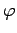, propagating in the positive-direction, is represented by the following wavefunction: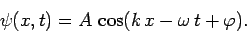(29)

Now, the type of wave represented above is conventionally termed a one-dimensional plane wave. It is one-dimensional because its associated wavefunction only depends on the single Cartesian coordinate. Furthermore, it is a plane wave because the wave maxima, which are located at(30)

where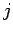is an integer, consist of a series of parallel planes, normal to the-axis, which are equally spaced a distance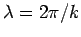apart, and propagate along the positive-axis at the velocity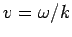. These conclusions follow because Eq. (30) can be re-written in the form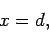(31)

where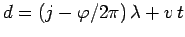. Moreover, as is well-known, (31) is the equation of a plane, normal to the-axis, whose distance of closest approach to the origin is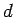.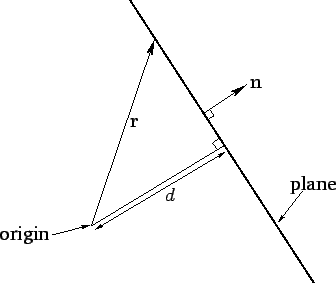The previous equation can also be written in the coordinate-free form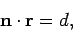(32)

where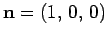is a unit vector directed along the positive-axis, andrepresents the vector displacement of a general point from the origin. Since there is nothing special about the-direction, it follows that if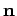is re-interpreted as a unit vector pointing in an arbitrary direction then (32) can be re-interpreted as the general equation of a plane. As before, the plane is normal to, and its distance of closest approach to the origin is. See Fig. 1. This observation allows us to write the three-dimensional equivalent to the wavefunction (29) as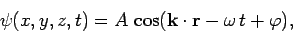(33)

where the constant vector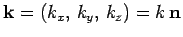is called the wavevector. The wave represented above is conventionally termed a three-dimensional plane wave. It is three-dimensional because its wavefunction,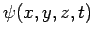, depends on all three Cartesian coordinates. Moreover, it is a plane wave because the wave maxima are located at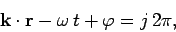(34)

or(35)

where, and. Note that the wavenumber,, is the magnitude of the wavevector,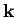: i.e.,. It follows, by comparison with Eq. (32), that the wave maxima consist of a series of parallel planes, normal to the wavevector, which are equally spaced a distance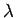apart, and which propagate in the-direction at the velocity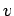. See Fig. 2. Hence, the direction of the wavevector specifies the wave propagation direction, whereas its magnitude determines the wavenumber,, and, thus, the wavelength,.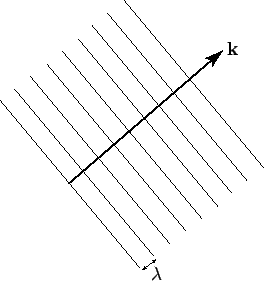Next: Representation of Waves via Up: Wave-Particle Duality Previous: Wavefunctions
Richard Fitzpatrick 2010-07-20